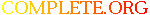Complete.Org: Mailing Lists: Archives: freeciv-ai: May 2004: [freeciv-ai] (PR#8740) segmentation fault in ai_hunter_findjob# [freeciv-ai] (PR#8740) segmentation fault in ai_hunter_findjob

[Top] [All Lists]

 To: undisclosed-recipients: ; Subject: [freeciv-ai] (PR#8740) segmentation fault in ai_hunter_findjob From: "Vasco Alexandre da Silva Costa" Date: Fri, 14 May 2004 14:49:03 -0700 Reply-to: rt@xxxxxxxxxxx

```<URL: http://rt.freeciv.org/Ticket/Display.html?id=8740 >

I keep finding bugs in the code. Interesting. :-)

Here is my latest catch:
Program received signal SIGSEGV, Segmentation fault.
0x080f20e8 in ai_hunter_findjob (pplayer=0x82a05a8, punit=0x9788c80)
at aihunt.c:302

(gdb) bt
#0  0x080f20e8 in ai_hunter_findjob (pplayer=0x82a05a8, punit=0x9788c80)
at aihunt.c:302
#1  0x080f1b21 in eval_hunter_want (pplayer=0x82a05a8, pcity=0x97e5f60,
choice=0x97e65f4, best_type=35, veteran=0) at aihunt.c:191
#2  0x080f1c12 in ai_hunter_choice (pplayer=0x82a05a8, pcity=0x97e5f60,
choice=0x97e65f4) at aihunt.c:223
#4  0x080efccb in ai_manage_cities (pplayer=0x82a05a8) at aicity.c:515
#5  0x080f1668 in ai_do_last_activities (pplayer=0x82a05a8) at aihand.c:319
#6  0x0804dbf1 in end_turn () at srv_main.c:493
#7  0x0804eea6 in main_loop () at srv_main.c:1411
#8  0x0804eff6 in srv_main () at srv_main.c:1499
#9  0x0804a118 in main (argc=3, argv=0xfeee2554) at civserver.c:161

(gdb) print *ptile
\$5 = {terrain = T_OCEAN, special = S_NO_SPECIAL, city = 0x0, units =
{list = {
nelements = 1, null_link = {next = 0xf6b37dc0, prev = 0xf6b37dc0,
known = 255, assigned = -5, worked = 0x0, continent = 0,
move_cost = "ýýýýýýýý", owner = 0x0, client = {hilite = HILITE_NONE},
spec_sprite = 0x0}

This should be the target I guess:
\$6 = {type = 43, id = 1506, owner = 2, x = 5, y = 71, homecity = 225,
moves_left = 0, hp = 30, veteran = 0, unhappiness = 0, upkeep = 0,
upkeep_food = 0, upkeep_gold = 0, fuel = 0, bribe_cost = -1, ai = {
control = false, ai_role = AIUNIT_NONE, ferryboat = 0, passenger = 0,
bodyguard = 0, charge = 0, prev_struct = {x = 0, y = 0}, cur_struct = {
x = 0, y = 0}, prev_pos = 0x0, cur_pos = 0x0, target = 0, hunted = 0},
activity = ACTIVITY_IDLE, goto_dest = {x = 23, y = 59}, activity_count
= 0,
activity_target = S_NO_SPECIAL, focus_status = FOCUS_AVAIL, ord_map = 0,
ord_city = 0, foul = false, debug = false, moved = true,
paradropped = false, connecting = false, done_moving = true,
transported_by = -1, occupy = 0, has_orders = false, orders = {length = 0,
index = 0, repeat = false, vigilant = false, list = 0x0}}

Here is punit:
(gdb) print *punit
\$7 = {type = 35, id = 0, owner = 0, x = 92, y = 65, homecity = 1303,
moves_left = 12, hp = 20, veteran = 0, unhappiness = 0, upkeep = 0,
upkeep_food = 0, upkeep_gold = 0, fuel = 0, bribe_cost = -1, ai = {
control = false, ai_role = AIUNIT_NONE, ferryboat = 0, passenger = 0,
bodyguard = 0, charge = 0, prev_struct = {x = 0, y = 0}, cur_struct = {
x = 0, y = 0}, prev_pos = 0x0, cur_pos = 0x0, target = 0, hunted = 0},
activity = ACTIVITY_IDLE, goto_dest = {x = -1, y = -1}, activity_count
= 0,
activity_target = S_NO_SPECIAL, focus_status = FOCUS_AVAIL, ord_map = 0,
ord_city = 0, foul = false, debug = false, moved = false,
paradropped = false, connecting = false, done_moving = false,
transported_by = -1, occupy = 0, has_orders = false, orders = {length = 0,
index = 0, repeat = false, vigilant = false, list = 0x0}}

I assume it crashes because here:
/* We can't chase if we aren't faster or on intercept vector */
if (unit_type(punit)->move_rate < unit_type(target)->move_rate
&& dist1 >= dist2) {
UNIT_LOG(LOGLEVEL_HUNT, punit, "can't get to %s(%d, %d) was (%d,
%d)",
unit_type(target)->name, target->x, target->y,
target->ai.prev_pos->x, target->ai.prev_pos->y);
continue;
}

target->ai.prev_pos is NULL and you are unreferencing it.

```